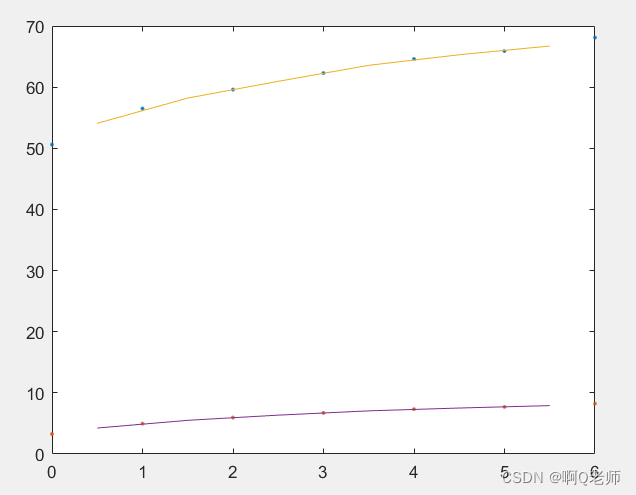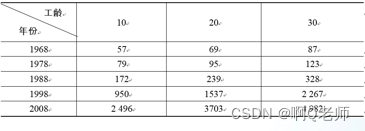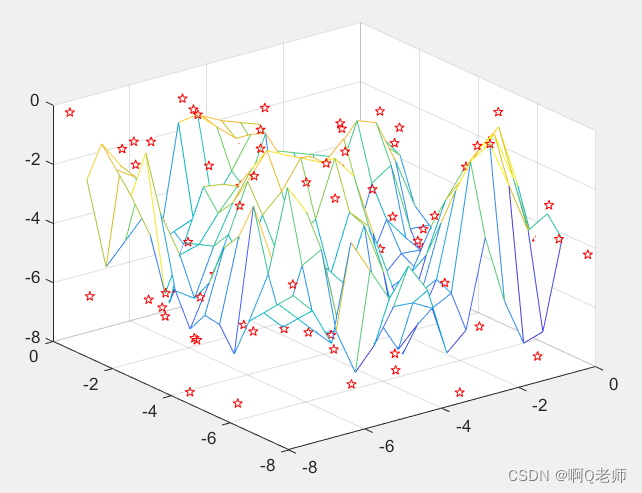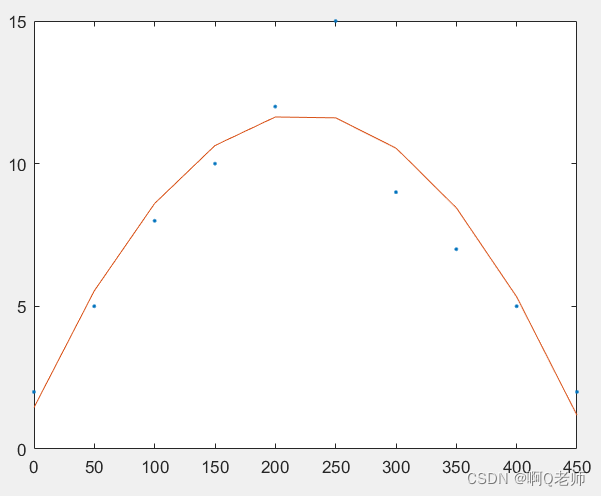STM32CubeMX

2021数模国赛

# 数据分析

## 求最大值与最小值

max函数用于对数据序列求最大值；min函数用于求一个数据序列的最小值，其用法与max函数相同。

jdk动态代理使用

Number_max = max(x)，其中，若x是向量，则返回向量X的最大值；若x是矩阵，则返回一个包含每一列最大值的行向量。
[Number_max,U] = max(x,[],dim)，dim表示维数，沿维度dim返回最大值。即如果x是一个矩阵的话，dim取1表示找这个矩阵中各列的最大值，dim取2表示找这个矩阵中各行的最大值。

airtest

``````%示例：

>> x = [15 18 98;102 913 202;25 68 75];
>> Number_max = max(x); %求矩阵x中各列元素的最大值
>> Number_min = min(x); %求矩阵x中各列元素的最小值
>> Number_max2 = max(x,[],2); %求矩阵x中各行元素的最大值
>> disp(Number_max)
102   913   202

>> disp(Number_min)
15    18    75

>> disp(Number_max2)
98
913
75
``````

max(x,y)：返回值是与x、y同型的向量或矩阵，其中的每个元素为x、y对应元素的较大者。
max(x,n)：n是一个标量，返回值是与x同型的向量或矩阵，其中的每个元素为x对应元素或n的较大者。

``````%示例：
>> x = [15 18 98;102 913 202;25 68 75];
>> y = [98 23 19;23 88 80;12 88 99];
>> max(x,y)

ans =

98    23    98
102   913   202
25    88    99

>> n = 68; %标量
>> min(x,n)

ans =

15    18    68
68    68    68
25    68    68
``````

## 求平均值与中值

mean函数用于对数据序列求平均值；median函数用于对数据序列求中值，其用法与mean函数相同。

m = mean(x)，其中，如果x是一个向量，则返回向量的算术平均值；如果x是一个矩阵，则返回一个行向量，其第i个元素是x的第i列的算术平均值。
m = mean(x,dim)，其中，当dim为1（默认值）时，该函数等同于mean(x)；当dim为2时，返回一个列向量，其第i个元素是x的第i行的算术平均值。

ceil向上取整

``````%示例：
>> x = 0:1:8;
>> m = [mean(x),median(x)]

m =

4     4
``````

## 求和与积

sum函数用于对数据序列求和；prod函数用于对数据序列求积，其用法与sum函数相同。

s = sum(x)，其中，如果x是一个向量，则返回向量各元素的和；如果x是一个矩阵，则返回一个行向量，其第i个元素是x的第i列的元素和。
s = sum(x,dim)，其中，当dim为1（默认值）时，该函数等同于sum(x)；当dim为2时，返回一个列向量，其第i个元素是x的第i行的各元素之和。

``````%示例：
>> x = [1 2 3;4 5 6;7 8 9];
>> s1 = sum(x)

s1 =

12    15    18

>> s2 = sum(x,2)

s2 =

6
15
24

>> p1 = prod(x)

p1 =

28    80   162

>> p2 = prod(x,2)

p2 =

6
120
504
``````

## 求累加和与累乘积

cumsum函数用于求累加和；cumprod函数用于求累乘积，其用法与cumsum函数相同。

s = cumsum(x)：如果x是一个向量，则返回累加和向量；如果x是一个矩阵，返回的矩阵的第i列是x的第i列的累加和向量。
x = cumsum(x,dim)：返回多维数组的累加和。若x是矩阵，当dim为1（默认值）时，返回的矩阵的第i列是x的第i列的累加和向量；当dim为2时，返回的矩阵的第i行是x的第i行的累加和向量。

CSDN开发云

``````%示例：
>> %求S=1+（1+3）+(1+3+5)+…+(1+3+…+11)的值。
>> x = 1:2:11;
>> y = cumsum(x)

y =

1     4     9    16    25    36

>> s = sum(y)

s =

91
``````

## 统计描述函数

LSTNet

### 标准差

std函数用来计算标准差。对于向量x，std(x)返回一个标量。对于矩阵x，std(x)返回一个行向量，它的各个元素便是矩阵x各列或各行的标准差。

s = std(x , w, dim)，其中，选项w用于指定标准差的计算方法，当w为0（默认值，一般都为0）时，表示求标准差时除n-1；当w为1时，表示求标准差时除n。选项dim为1（默认值）时，求各列元素的标准差；dim为2时，求各行元素的标准差。

``````%示例：
>> x = [7,4,9,8,10,7,8,7,8,7;7,6,10,5,9,8,10,9,5,6];
>> std(x,0,2) %求各行元素的标准差时除以n-1。

ans =

1.5811
1.9579
``````

### 方差

var函数用来计算方差。对于向量x，var(x)返回一个标量。对于矩阵x，var(x)返回一个行向量，各个元素是矩阵x各列或各行的方差。

v = var(x, w, dim)，其中，选项w用于指定权重方案。若w为0（默认值），则按观测值数量-1（即k=n-1）实现归一化；若w为1，则按观测值数量（即k=n）实现归一化。选项dim指定沿维度dim计算方差。对于矩阵，当dim 为1（默认值）时，求各列元素的方差；当dim为2时，求各行元素的方差。

``````%示例：
>> x = [7,4,9,8,10,7,8,7,8,7;7,6,10,5,9,8,10,9,5,6];
>> v = var(x,0,2)

v =

2.5000
3.8333
``````

### 相关系数

corrcoef函数计算数据的相关系数。

[R,P] = corrcoef(X,Y)，其中，返回相关系数矩阵和p值矩阵。如果得到的p值矩阵的非对角线元素小于显著性水平（即90%置信区间，默认为 0.05），则R中的相应相关性被视为显著。
[R,P] = corrcoef(X)，其中，返回矩阵X各列的相关系数，计算时把矩阵X的每列作为一个观测变量，然后求各列的相关系数。

### 协方差

cov函数用于计算数据序列的协方差。

c = cov(x)，其中，若x是向量，则返回x的方差；若x是矩阵，则x的每一行代表一个样本，每一列代表一个观测变量。
c = cov(x,y)，其中，返回变量x与y的协方差，x与y同样大小。如果x与y是矩阵，则cov(x,y) 将x与y视为列向量，等价于 cov(x( : ), y( : ))。

``````%示例：随机抽取15名健康成人，测定血液的凝血酶浓度及凝血时间，分析凝血酶浓度与凝血时间之间的相关性。
>> x = [1.1,1.2,1.0,0.9,1.2,1.1,0.9,0.6,1.0,0.9,1.1,0.9,1.1,1,0.7];
>> y = [14,13,15,15,13,14,16,17,14,16,15,16,14,15,17];
>> r = corrcoef(x,y)

r =

1.0000   -0.9265
-0.9265    1.0000

>> c = cov(x,y)

c =

0.0289   -0.2014
-0.2014    1.6381
``````

## 排序

sort函数用于对数组元素进行排序。

[y,i] = sort(x, dim, mode)，其中，y是排序后的矩阵，而i记录y中的元素在x中的位置。

``````%示例：
>> A = [2 3 1;6 5 4;7 9 8];
>> y = sort(A,2)

y =

1     2     3
4     5     6
7     8     9
``````

# 多项式计算

## 多项式的加减运算

``````%示例：
>> %求(2.*x.^3+8.*x.^2-5.*x+8)+(6.*x.^2+5.*x+10)
>> a = [2 8 -5 8]; %对应系数
>> b = [0 6 5 10];
>> c = a + b

c =

2    14    0    18

%即为2.*x.^3+14.*x.^2+18
``````

## 多项式的乘除运算

conv函数用于计算多项式的乘积运算；deconv函数用于计算多项式的除法运算。

w = conv(p1,p2)
[Q,r] = deconv(p1,p2)

``````%示例：
>> %求(2.*x.^4+8.*x.^3-8)x(2.*x.^2-3.*x) 与 x.^3-x.^2/x.^2-x
>> p1 = [2 8 0 0 -8];
>> p2 = [2 -3 0];
>> c = conv(p1,p2)

c =

4    10   -24     0   -16    24     0

>> p3 = [1 -1 0 0];
>> p4 = [1 -1 0];
>> [P,r] = deconv(p3,p4)

P =

1     0

r =

0     0     0     0
``````

## 多项式的求导

k = polyder( P )：求多项式P的导数；
k = polyder(P,Q)：求P·Q的导数；
[q,d] = polyder(P,Q)：求P/Q的导数。

rapidio

``````%示例：
%记f(x) = (x-1)(x-2)...(x-n),g(x)=(x+1)(x+2)...(x+n)，
%则f'(x) = (x-1)'(x-2)(x-3)...(x-n)+(x-1)(x-2)'(x-3)...(x-n)+...+(x-1)(x-2)...(x-n)'；
%记f(x) = h(x)/g(x)，
%f'(x) = (g(x)*h'(x)-h(x)*g'(x))/(g(x))^2.
>> %求多项式2.*x.^3 + 4.*x.^2 + 6.*x + 8的导数
>> p = [2 4 6 8];
>> k = polyder(p)

k =

6     8     6

>> %求多项式1/(x.^2+8)的导数
>> P = ;
>> Q = [1 0 8];
>> [p,q]=polyder(P,Q)

p =

-2     0

q =

1     0    16     0    64
``````

## 多项式的求值

polyval函数用来求代数多项式的值。

y = polyval(p,x)，其中，p是多项式系数向量。若x为标量，得到多项式在该点的值；若x为向量或矩阵，则对向量或矩阵中的每个元素求多项式的值。

``````%示例：
>> %已知多项式8.*x.6 + 6.*x.^4 - 7.*x.^2 + 10，当x=8时，该多项式的值
>> A = [8 0 6 0 -7 0 10];
>> x = 8;
>> y = polyval(A,x)

y =

2121290
``````

## 多项式的求根

roots函数用于求多项式的全部根。

x = roots( p )，其中，p为多项式的系数向量，求得的根赋给向量x，即x(1)，x(2)，…，x(n)分别代表多项式的n个根。

emmc

``````%示例：
>> %已知f(x) = x.^2 + 2.*x + 1，求f(x)=0的全部根。
>> p = [1 2 1];
>> x = roots(p)

x =

-1
-1
``````

# 数据插值

unity

## 一维数据插值

interp1函数用于实现一维插值的。

vq = interp1(x,v,xq,method,extrapolation)

xq被插值点，vq是在被插值点xi处的插值结果，是一个列的长度与xq相同、宽度与v相同的矩阵。

'nearest’是最邻近插值； 'linear’线性插值（默认值）； 'spline’三次样条插值； 'cubic’立方插值。

``````%示例：
>> %用3次样条插值分别求得婴儿出生后半个月到5个半月每隔1个月的身长、体重参考值。
>> %0~6个月婴儿的体重、身长参考标准：
>> %时间：0 1 2 3 4 5 6
>> %体重：3.27 4.97 5.95 6.73 7.32 7.7 8.22
>> %身长：50.6 56.5 59.6 62.3 64.6 65.9 68.1
>> x = 0:1:6;
>> v = [50.6,3.27; 56.5,4.97; 59.6,5.95; 62.3,6.73; ...
64.6,7.32; 65.9,7.70; 68.1,8.22];
>> xq = 0.5:1:5.5;
>> vq = interp1(x,v,xq,'spline')

vq =

54.0847    4.2505
58.2153    5.5095
60.9541    6.3565
63.5682    7.0558
65.2981    7.5201
66.7269    7.9149

>> %并绘制图形:
>> plot(x,v,'.',xq,vq)
``````NeRF

## 多维数据插值

interp2函数用于实现二维插值的。

zq = interp2(x, y, v, xq, yq, method, extrapval)

xq、yq存储插值点的平面坐标，zq是根据相应的插值方法得到的插值点的值。

openmv

vq = interp3(x, y, z, v, xq, yq, zq, method)
vq = interpn(x1, x2,…,xn, v, xq1, xq2,…,xqn, method)``````%题解：
x = [10 20 30];
y = [1968:10:2008]'; %转置
v = [57 69 87;
79 95 123;
172 239 328;
950 1537 2267;
2496 3703 4982];
xq = [15 25];
yq = [1973:5:2003]'; %转置
zq = interp2(x, y, w, xq, yq, 'linear')

zq =

1.0e+03 *

0.0750    0.0935
0.0870    0.1090
0.1462    0.1963
0.2055    0.2835
0.7245    1.0928
1.2435    1.9020
2.1715    3.1223

%答案为（1.0e+03 *）十的三次方乘以每位小数，即75 935 ......
``````

## 散乱数据插值

griddata函数实现散乱数据插值。

vq = griddata(x, y, v, xq, yq, method)
vq = griddata(x, y, z, v, xq, yq, zq, method)

xq、yq、zq存储插值点的坐标，vq是根据相应的插值方法得到的插值结果。

'nearest’是最邻近插值； 'linear’线性插值（默认值）； 'natural’自然邻域插值； 'cubic’立方插值；'v4’双调和样条插值。

``````%示例：
%随机生成包含100个散点的数据集，绘制散点数据图和插值得到的网格数据图，观察插值结果。
xy = rand(100,3)*8-8; %生成一个100x3的随机矩阵（数值范围在0~1之间），然后每个数乘上8后再减8，最后得出结果
x = xy(:,1); %获取xy第一列所有元素
y = xy(:,2); %获取xy第二列所有元素
z = xy(:,3); %获取xy第三列所有元素
a = -8:0.5:8;
[xq,yq] = meshgrid(a); %生成二维网格坐标矩阵
zq = griddata(x,y,z,xq,yq);
mesh(xq,yq,zq) %绘制三维网格图
hold on %叠图
plot3(x,y,z,'rp')
``````# 曲线拟合

polyfit函数用于求最小二乘拟合多项式的系数。

p = polyfit(x,y,n)
[p,s] = polyfit(x,y,n)
[p,s,mu] = polyfit(x,y,n)

``````%示例：
>> %研究水量对白菜生长的影响，做了十次实验。分析其关系
>> %水量：0 50 100 150 200 250 300 350 400 450
>> %相同情况下的白菜生长情况：2 5 8 10 12 15 9 7 5 2
>> data = [0 2; 50 5; 100 8; 150 10; 200 12; 250 15; 300 9; 350 7; 400 5; 450 2];
>> x = data(:,1)
>> x = data(:,1);
>> y = data(:,2);
>> f = polyfit(x,y,2); %返回阶数为2的多项式f(x)的系数
>> yi = polyval(f,x); %求在x处的n阶多项式的值
>> plot(x,y,'.',x,yi)
``````# 非线性方程和非线性方程组的数值求解

fzero函数用来求非线性方程的根。

x = fzero(@fun, x0, options)
[x, fval, exitflag, output] = fzero(@fun, x0, options)

``````%示例：
>> %求exp(-2.*x)-x = 0在x0 = 0附近的根
>> x1 = fzero(@(x)exp(-2.*x)-x,0)

x1 =

0.4263
``````

fsolve函数用于求非线性方程组F(x) = 0的数值解。

x = fsolve(fun,x0)

``````%示例：
>> %求非线性方程组在(1,1)附近的数值解。x1.^2 + x1 - x2.^2 = 1 与 x2 - sin(x1.^2) = 0
>> %先建立函数文件

function y = func(f)
x1 = f(1);
x2 = f(2);
y(1) = x1.^2 + x1 - x2.^2 - 1;
y(2) = x2 - sin(x1.^2);

>> %保存并返回命令窗口
>> %再调用fsolve函数求解
>> x0 = [1;1];
>> x = fsolve(@func,x0)

x =

0.7260
0.5029
``````